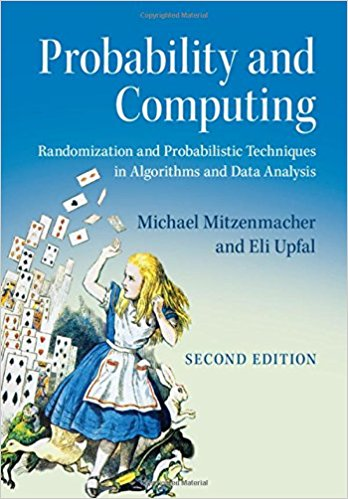E-BOOK

# Probability and Computing: Randomization and Probabilistic Techniques in Algorithms and Data AnalysisRandomization and probabilistic techniques play an important role in modern computer science, with applications ranging from combinatorial optimization and machine learning to communication networks and secure protocols. This 2005 textbook is designed to accompany a one- or two-semester course for advanced undergraduates or beginning graduate students in computer science and applied mathematics. It gives an excellent introduction to the probabilistic techniques and paradigms used in the development of probabilistic algorithms and analyses. It assumes only an elementary background in discrete mathematics and gives a rigorous yet accessible treatment of the material, with numerous examples and applications. The first half of the book covers core material, including random sampling, expectations, Markov’s inequality, Chevyshev’s inequality, Chernoff bounds, the probabilistic method and Markov chains. The second half covers more advanced topics such as continuous probability, applications of limited independence, entropy, Markov chain Monte Carlo methods and balanced allocations. With its comprehensive selection of topics, along with many examples and exercises, this book is an indispensable teaching tool.

Probability and Computing 2nd Edition